CBSE Class 12 Sample Paper for 2024 Boards

Class 12
Solutions of Sample Papers and Past Year Papers - for Class 12 Boards

## If f(x)=1/(4x^2  + 2x + 1);x∈R, then find the maximum value of f(x).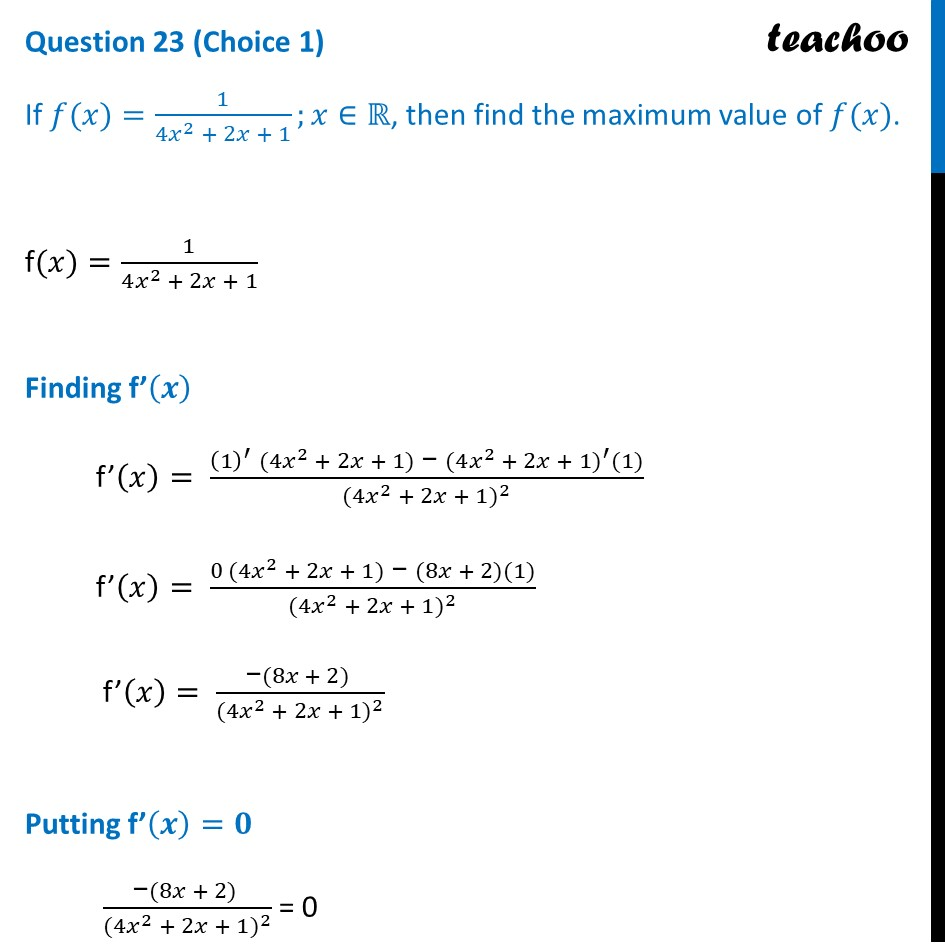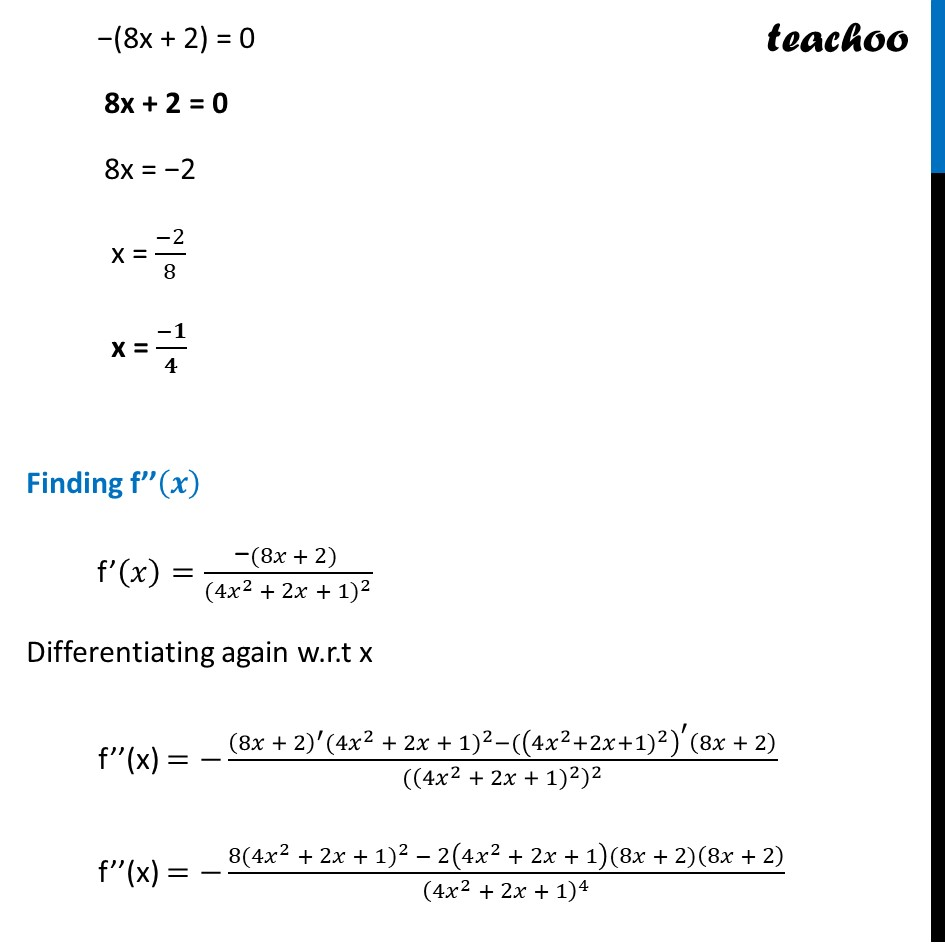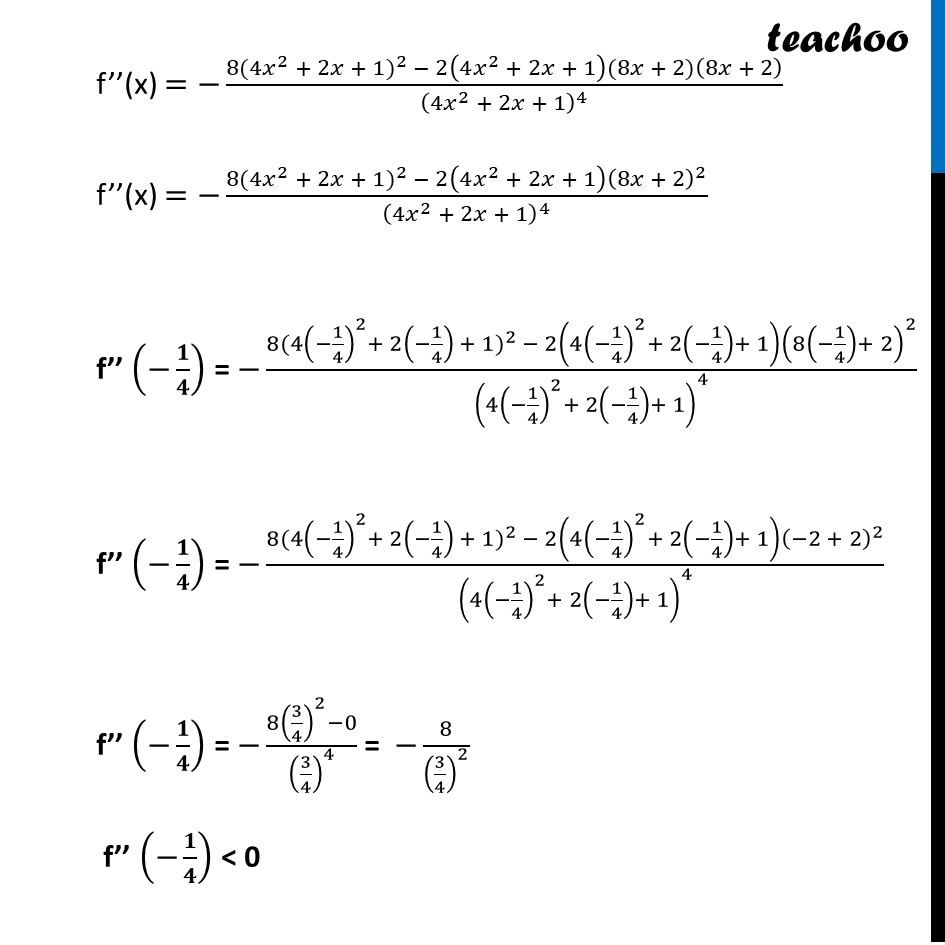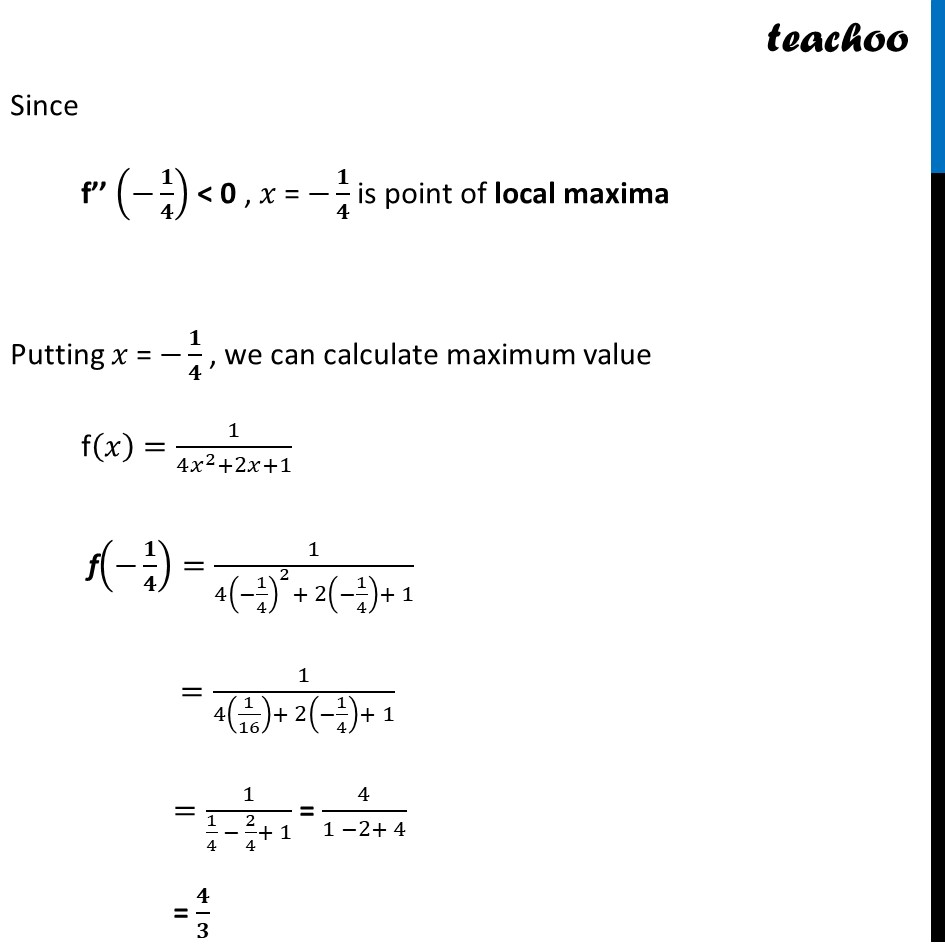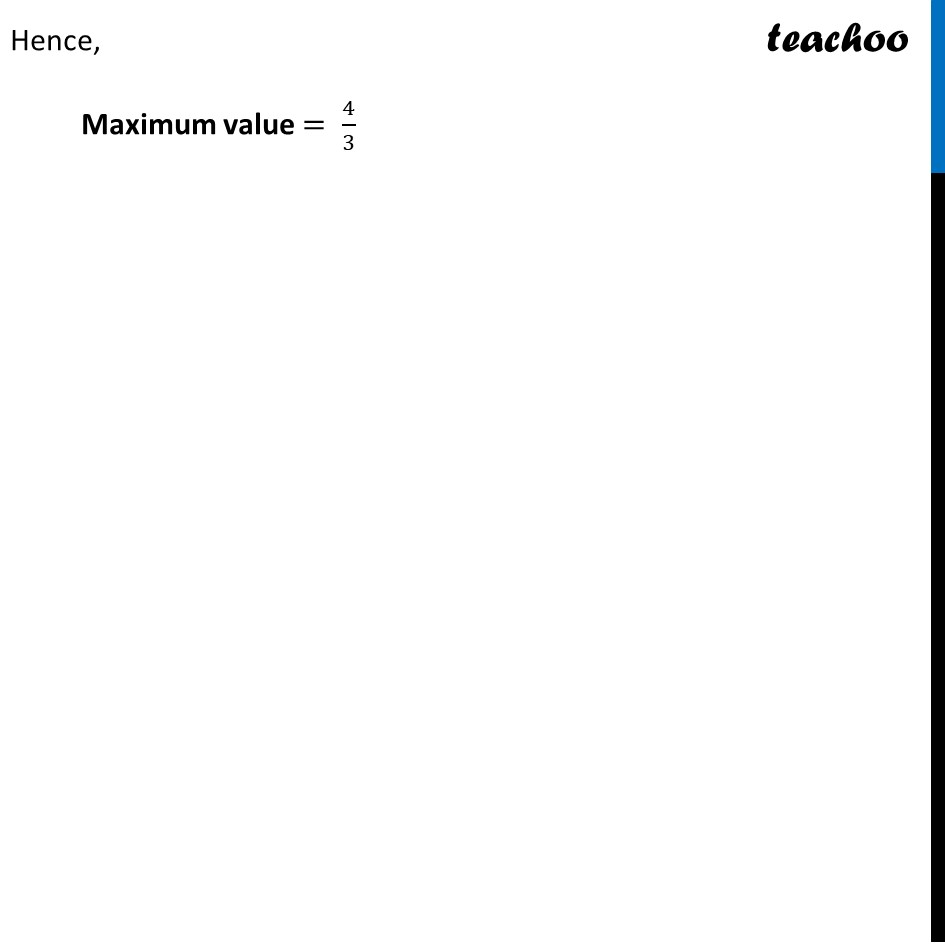Learn in your speed, with individual attention - Teachoo Maths 1-on-1 Class

### Transcript

f(𝑥)=1/(4𝑥^2 + 2𝑥 + 1) Finding f’(𝒙) f’(𝑥)= ((1)^′ " " (4𝑥^2 + 2𝑥 + 1)" − " (〖4𝑥^2 + 2𝑥 + 1)〗^′ (1))/((〖4𝑥^2 + 2𝑥 + 1)〗^2 ) f’(𝑥)= (0 (4𝑥^2 + 2𝑥 + 1)" − " (8𝑥 + 2)(1))/((〖4𝑥^2 + 2𝑥 + 1)〗^2 ) f’(𝑥)= ("−" (8𝑥 + 2) )/((〖4𝑥^2 + 2𝑥 + 1)〗^2 ) Putting f’(𝒙)=𝟎 ("−" (8𝑥 + 2) )/((〖4𝑥^2 + 2𝑥 + 1)〗^2 ) = 0 -(8x + 2) = 0 8x + 2 = 0 8x = -2 −(8x + 2) = 0 8x + 2 = 0 8x = −2 x = (−2)/8 x = (−𝟏)/𝟒 Finding f’’(𝒙) f’(𝑥)=("−" (8𝑥 + 2) )/((〖4𝑥^2 + 2𝑥 + 1)〗^2 ) " " Differentiating again w.r.t x f’’(x) =−((8𝑥 + 2)^′ (〖4𝑥^2 + 2𝑥 + 1)〗^2−((〖4𝑥^2+2𝑥+1)〗^2 )^′ (8𝑥 + 2))/(((〖4𝑥^2 + 2𝑥 + 1)〗^2 )^2 ) f’’(x) =−(8(〖4𝑥^2 + 2𝑥 + 1)〗^2 − 2(4𝑥^2 + 2𝑥 + 1)(8𝑥 + 2)(8𝑥 + 2))/(4𝑥^2 + 2𝑥 + 1)^4 f’’(x) =−(8(〖4𝑥^2 + 2𝑥 + 1)〗^2 − 2(4𝑥^2 + 2𝑥 + 1)(8𝑥 + 2)(8𝑥 + 2))/(4𝑥^2 + 2𝑥 + 1)^4 f’’(x) =−(8(〖4𝑥^2 + 2𝑥 + 1)〗^2 − 2(4𝑥^2 + 2𝑥 + 1) (8𝑥 + 2)^2)/(4𝑥^2 + 2𝑥 + 1)^4 f’’ (−𝟏/𝟒) = −(8(〖4(−1/4)^2+ 2(−1/4) + 1)〗^2 − 2(4(−1/4)^2+ 2(−1/4)+ 1) (8(−1/4)+ 2)^2)/(4(−1/4)^2+ 2(−1/4)+ 1)^4 f’’ (−𝟏/𝟒) = −(8(〖4(−1/4)^2+ 2(−1/4) + 1)〗^2 − 2(4(−1/4)^2+ 2(−1/4)+ 1) (−2 + 2)^2)/(4(−1/4)^2+ 2(−1/4)+ 1)^4 f’’ (−𝟏/𝟒) = −(8(3/4)^2−0)/(3/4)^4 = −8/(3/4)^2 f’’ (−𝟏/𝟒) < 0 Since f’’ (−𝟏/𝟒) < 0 , 𝑥 = −𝟏/𝟒 is point of local maxima Putting 𝑥 = −𝟏/𝟒 , we can calculate maximum value f(𝑥) =1/(4𝑥^2+2𝑥+1) f(−𝟏/𝟒)=1/(4(−1/4)^2+ 2(−1/4)+ 1) =1/(4(1/16)+ 2(−1/4)+ 1) =1/(1/4 − 2/4+ 1) = 4/(1 −2+ 4) = 𝟒/𝟑